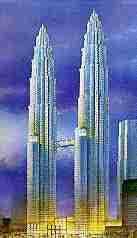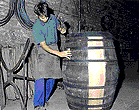Search IntMath
Close

450+ Math Lessons written by Math Professors and Teachers

5 Million+ Students Helped Each Year

1200+ Articles Written by Math Educators and Enthusiasts

Simplifying and Teaching Math for Over 23 Years

# Integration

## Why do we need to study Integration?

by M. BourneThe Petronas Towers
of Kuala Lumpur

Often we know the relationship involving the rate of change of two variables, but we may need to know the direct relationship between the two variables. For example, we may know the velocity of an object at a particular time, but we may want to know the position of the object at that time.

To find this direct relationship, we need to use the process which is opposite to differentiation. This is called integration (or antidifferentiation).

The processes of integration are used in many applications.

The Petronas Towers in Kuala Lumpur experience high forces due to winds. Integration was used to design the building for strength.Sydney Opera House

The Sydney Opera House is a very unusual design based on slices out of a ball. Many differential equations (one type of integration) were solved in the design of this building.Historically, one of the first uses of integration was in finding the volumes of wine-casks (which have a curved surface).

We learn to find the volume of these objects later (in volume of solid of revolution).

Other uses of integration include finding areas under curved surfaces, centres of mass, displacement and velocity, fluid flow, modelling the behaviour of objects under stress, etc.

## In this Chapter

### Related Sections in Interactive Mathematics

The Introduction to Calculus has a brief history of calculus.

Methods of Integration, which shows more advanced techniques for integrating.

Applications of Integration, which demonstrates how to solve several problems using integration.

Fourier Series and Fast Fourier Transform show the use of integration in the analysis of electronics.

Differential Equations are a special type of integration.

Laplace Transform of an integral is an important process in electronics.

We begin with a discussion of the differential, because it involves some of the concepts and notation used in the study of integration. The Differential »

## Problem SolverThis tool combines the power of mathematical computation engine that excels at solving mathematical formulas with the power of GPT large language models to parse and generate natural language. This creates math problem solver thats more accurate than ChatGPT, more flexible than a calculator, and faster answers than a human tutor. Learn More.